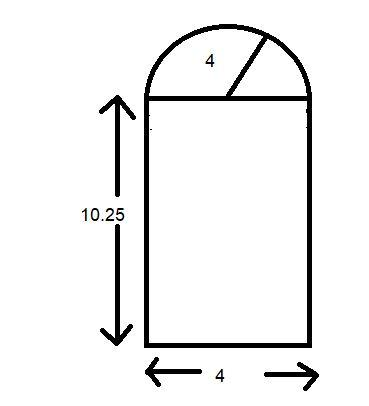Courses
Courses for Kids
Free study material
Free LIVE classes
More# A door is in the shape of a rectangle surmounted by a semicircle. If the width of the door is 4 units and the height of the door is 10.25 units, find the total area of the door.

Last updated date: 17th Mar 2023
Total views: 304.2k
Views today: 2.82kVerified
304.2k+ views
Hint: - Here we go through by first figure out the diagram what the question says then apply the formula of area of rectangle and the area of semicircle. And then add them together to find the total area of the door.Here it is given that the width of the door is 4 on which the semicircular is surmounted. By the figure we can say that the length of the width is equal to the diameter of the semi-circle.
Hence the radius of the semicircle ‘r’ is $\dfrac{4}{2} = 2$ because the radius is half of the diameter.
Given width of door =4.
Length of door =10.25.
And the door is surmounted by a semicircle of radius =2.
First we find out the area of rectangular figure by the help of the formula of area of rectangle i.e. $l \times b$
Area of rectangular figure =$l \times b = 4 \times 10.25 = 41$sq. unit
Now we find out the area of semicircle that is surmounted on the rectangular part by the formula of area of semicircle i.e. $\dfrac{{\pi {r^2}}}{2}$
Area of semicircular figure=$\dfrac{{\pi {r^2}}}{2} = \dfrac{{22}}{7} \times \dfrac{1}{2} \times 2 \times 2 = 6.28$ sq. unit
Now for the total area of the door we add the area of the rectangular part with the area of the semicircular part. I.e. 41+6.28=47.28 sq. unit.

Note: - Whenever we face such a type of question the key concept for solving the question is first figure out the diagram then apply the formula of area to find out the area of the given figure. And always remember one thing in mind that the unit of area is always in the form of sq. unit here in this question no unit is given to the question so we simply write its unit as sq. unit to show that is the value of area.